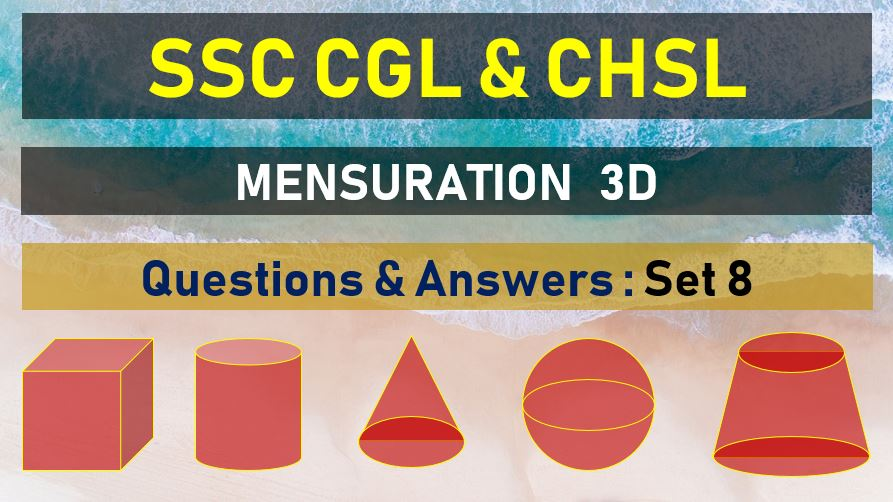Tuesday, June 22, 2021
Home > mensuration > SSC CHSL Mensuration 3D Questions Answers Set 8

# SSC CHSL Mensuration 3D Questions Answers Set 8SSC Mensurations 3D
Surface Area & Volume Questions Sets
Set 1Set 2Set 3Set 4Set 5
Set 6Set 7Set 8Set 9Set 10

SSC CHSL Mensuration 3D Questions Answers Set 8: Ques No 1

If each edge of a cube is increased by 50% the percentage increase in its surface area is

Options:

A. 75 %

B. 100 %

C. 125 %

D. 150 %

Answer: C

Solution:

SSC CHSL Mensuration 3D Questions Answers Set 8: Ques No 2

If the radius of a sphere be doubled the area of its surface will become

Options:

A. 2 times

B. 3 times

C. 4 times

D. 5 times

Answer: C

Solution:

SSC CHSL Mensuration 3D Questions Answers Set 8: Ques No 3

The height of a circular cylinder is increased six times and the base area is decreased to one ninth of its value. The factor by which the lateral surface of the cylinder increases is

Options:

A. 2

B. 1/2

C. 2/3

D. 3/2

Answer: A

Solution:

SSC CHSL Mensuration 3D Questions Answers Set 8: Ques No 4

A hemispherical cup of radius 4 cm is filled to the brim with coffee, The coffee is then poured into a vertical cone of radius 8 cm and height 16 cm. The percentage of the volume of the cone that remains empty is :

Options:

A. 87.5 %

B. 80.5 %

C. 81.6 %

D. 88.2 %

Answer: A

Solution:

SSC CHSL Mensuration 3D Questions Answer Set 8: Ques No 5

If the height of a cone is increase by 100% then its volume is increased by :

Options:

A. 100 %

B. 200 %

C. 300 %

D. 400 %

Answer: A

Solution:

SSC CHSL Mensuration 3D Questions Answer Set 8: Ques No 6

Each of the radius of the base and the height of a right circular cylinder is increased by 10%. The volume of the cylinder is increased by

Options:

A. 3.31 %

B. 14.5 %

C. 33.1 %

D. 19.5 %

Answer: C

Solution:

SSC CHSL Mensuration 3D Questions Answer Set 8: Ques No 7

The length, breadth and height of a cuboid are in the ratio 1 : 2: 3. If they are increased by 100%, 200% and 200% respectively. then compared to the original volume the increase in the volume of the cuboid will be

Options:

A. 5 times

B. 18 times

C. 12 times

D. 17 times

Answer: D

Solution:

SSC CHSL Mensuration 3D Questions Answer Set 8: Ques No 8

If the radius of a right circular cylinder is decreased by 50% and its height is increased by 60% its volume will be decreased by

Options:

A. 10 %

B. 60 %

C. 40 %

D. 50 %

Answer: B

Solution:

SSC CHSL Mensuration 3D Questions Answer Set 8: Ques No 9

If the radius of a sphere is doubled, its volume becomes

Options:

A. 2 times

B. 4 times

C. 6 times

D. 8 times

Answer: D

Solution:

SSC CHSL Mensuration 3D Questions Answer Set 8: Ques No 10

If the height of a cylinder is increased by 15 per cent and the radius of its base is decreased by 10 percent then by what percent will its curved surface area change?

Options:

A. 3.5 % decrease

B. 3.5 % increase

C. 5 % increase

D. 5 % decrease

Answer: B

Solution:

SSC CHSL Mensuration 3D Questions Answer Set 8: Ques No 11

A hollow iron pipe is 21 cm long and its external diameter is 8 cm. If the thickness of the pipe is 1 cm and iron weighs 8 g/cm3, then the weight of the pipe is:

Options:

A. 3.6 kg

B. 3.696 kg

C. 36 kg

D. 36.9 kg

Answer: B

SSC Mensurations 3D
Surface Area & Volume Questions Sets
Set 1Set 2Set 3Set 4Set 5
Set 6Set 7Set 8Set 9Set 10
error: Content is protected !!